output.to from Sideway
Draft for Information Only

# Content

`Virtual Work Work of a Force Work of a Couple Principle of Virtual Work Virtual Displacement Application of Principle of Virtual Work Mechanical Efficiency`

# Virtual Work

The unknown force acting on a rigid body is usually solved by balancing the external forces and moments acting on the body and solving a system of simultaneous equations using the equilibrium state of the rigid body. In mechanic, work is the displacement of a body done by a force or a moment. If there is no displacement of the body, then no work is done by a force acting on the body. By applying the principle of virtual work, used by Jean Bernoulli, the total virtual work of a body or a system of connected bodies in equilibrium is equal to zero. Therefore unknown force can be determined by applying a virtual displacement to the system of connected bodies.

## Work of a Force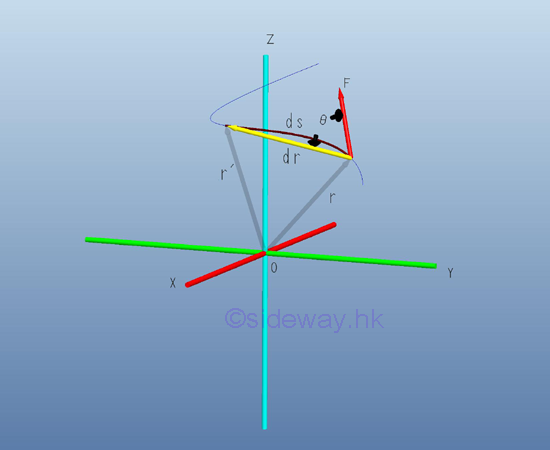By definition, work is done by a force when there is a displacement at the point of application in the line of action of the force with magnitude equal to the product of the applied force and the linear displace along the line of action of the force.  A displacement is the change of the position. A displacement of a body is the linear displacement of the body. An angular displacement is the change of the orietation by means of rotation. Although a finite angular displacement is not a vector quantity, the travelled distance due to an infinitesimal angular displacement can be used to approximate the corresponding displacement vector quantity. Therefore an infinitesimal work done by a force acting on a body with an differential displacement can be expressed as the scalar product of the force vector and the displacement vector. Imply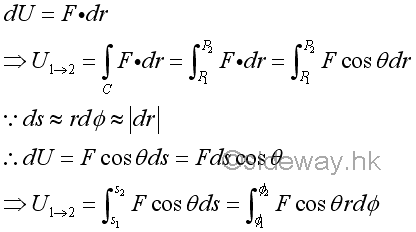In general work done by an applied force is dependent on the path C travelled. But for a conservative force, the total work done by a conservative force only depends on the displacement and is independent on the path C travelled. And for a non-conservative force, like friction, the total work done is dependent on the path travelled. However, the work done by an applied force undergoes infinitesimal angular displacement can be approximated as the work done by an applied force undergoes a linear displacement vector quantity.

By definition, the scalar product of two vectors is a scalar quantity of no direction with magnitude and sign only. Since the angle between the force vector and the displacement is from 0≤θ≤180o  , the value of work done is negative when the angle is 90o<θ≤180o . And if the force vector is perpendicular to the displacement, i.e angle=90o , then no work is done.When a rigid body or a system of rigid connected bodies is in equilibrium, both the external forces and the internal forces are in equilibrium. Besides, the total work of the internal forces is equal to zero also. For example, by imagining two points in a rigid body is connected by a link under two equal and opposite forces F and -F along the link. Although the displacement dr and dr' can be different, the components of these displacements along the axis of link must be equal because the distance between the two points must remain at the same distance from each other while moving by the forces.

## Work of a Couple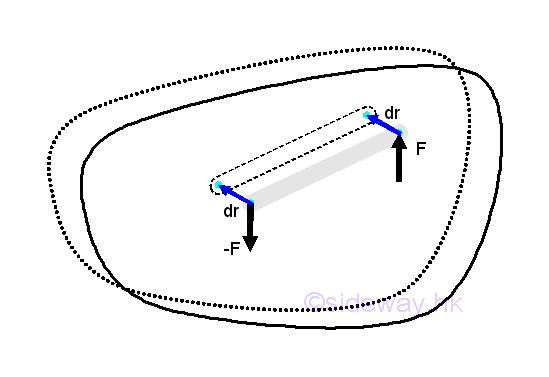If a rigid body is under a translation motion, the total work of a couple of forces acting on the rigid body is always equal to zero because there is no change in the orientation of the rigid body and the displacement of the particles are the same while the forces of a couple is always equal in magnitude and opposite in direction. ImplyHowever, when the couple of forces acting on the rigid body causes an infinitesimal angular displacement parallel to the applied forces couple, the total work of the couple of foces is then not equal to zero.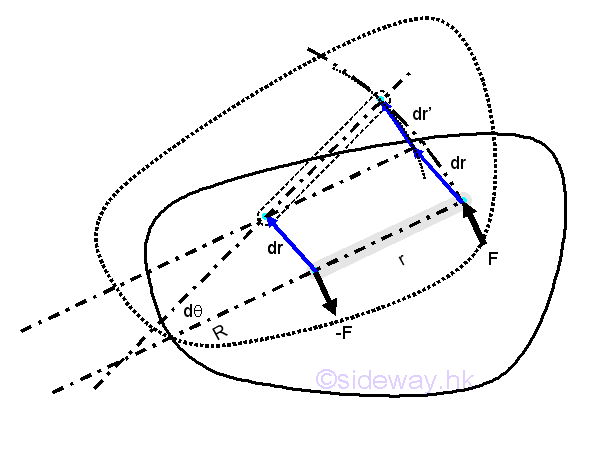The work of the force F can be divided into two parts, therefore the total work of a couple of forces is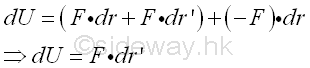Since the forces F and -F are always tangent to the arcs of rotation at the point of the application of force accordingly, therefore the total work of the couple of moment isSimilar to the work of a force, the instantaneous moment of the couple and the infinitesimal angular displacement should be in parallel, the work of a couple can be expressed as the dot product of two vector. ImplyAnd therefore the work of a couple is equal to zero if the moment vector is perpendicular to the infinitesimal angular displacement. And if the moment M acting on the rigid body is a constant and the finite angular displacement Δθ is in the same direction as the moment during the angular rotation in radian. Imply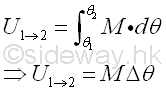## Principle of Virtual Work

Virtual work is the virtual displacement of a body done by a force or a moment acting on it. A displacement is the actual change of the position, while a virtual displacement is an imaginary differential displacement of a possible virtual displacement under actual displacement motion. And there are also linear and angular displacements. In other words, a possible virtual displacement acts as the simulation of a possible actual motion of the considered body under a first order differential displacement, at which the actual motion is possible to be performed. For example, a pinned linkage allows angular displacement while a weld linkage is fixed. Since the possible virtual displacement is to evaluate the work due to virtual displacement under the equilibrium state of the considered body, the possible compatible virtual displacement does not necessarily to be conformed to the constraints of the connections and supports of the considered body, because the constrains are already represented by the force vectors, which are used for the evaluation of the total virtual work of interested forces of the considered body under possible virtual displacement. Therefore both compatible with constrained virtual displacement or compatible virtual displacement can be used for detemined the virtual work. Usually, the virtual displacement is of first order accuracy with the second-order quantity can be neglected.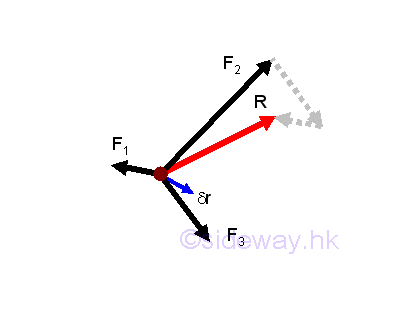Consider a virtual displacement of a particle, the virtual work of the particle done by forces acting on the particle will be equal to the sum of the virtual work done by each force. And therefore the total virtual work δU done on the particle is equal to the virtual work δU done by the resultant force R. Imply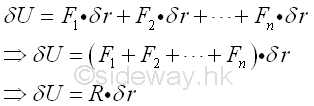Therefore if the particle is in equilibrium, the resultant force R should be equal to zero, and the work done by the resultant force on the particale is equal to zero also.In other words, when the work done on a particle is equal to zero for any virtual displacement δr, the particle must be in equilibrium or R is equal to zero. Imply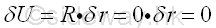Or, when a particle is in equilibrium, the total virtual work done by forces acting on the particle is zero for any virtual displacement of the particle because the resultant force acting on the particle is zero when the particle is in equilibrium.Since when a body is in equilibrium, all particles of the body must also be in equilibrium. Therefore the total virtual work done by forces acting on a particle is equal to zero when the particle is in equilibrium, the total virtual work done by all external and internal forces acting on all particles of the body for a compatible virtual displacement must also be zero when the body is in equilibrium. As the body is in equilibrium, the total virtual work done by all internal forces acting on all particle is zero, the total virtual work done by all external forces acting on the body must be zero also.Therefore the principle of virtual work in static is the making use of the property of total virtual work, which is equal to zero, for an equilibrium free body. When a body is in equilibrium state, the system of external forces acting on a free body is in equilibrium, and the total virtual work done by the system of external forces acting on the free body for a compatible virtual displacement is equal to zero. The free body can be a particle, a rigid body, a connected particles and a conneced rigid bodie, e.g. frame, machine.

## Virtual Displacement

Since the constraints provided by supports and connections in static equilibrium are used to define the reactions acting on the interested free body only, the virtual displacements of a free body are not limited by the constraints. However the virtual displaements of a free body are limited by the reaction forces acting on the free body and the compatibility of the allowable motions of the free body. Therefore virtual displacement of a body is an imaginary set of compatible differential displacements of first order with fixed relation in equilibrium state.For a first order accuracy differential displacement of a body, the second-order quantity can be neglected. The virtural linear displacement of the acting points on the free body can be related to a virtual angular displacement of the body which is assumed to be a rotation of the free body or the components of the free body about a displacement center. The relation between the virtual linear displacements of points on the free body can be determined approximately geometrically. For example, a ladder of length, l leans against a wall at an angle of θ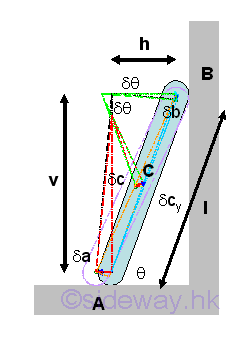Since the positions of points A, B, and C are fixed, the displacement centres of A and B, C and A, and C and B can be found by constructing the radius of rotation and therefore the relation between δa and δb, δc and δa, and δc and δb can also be determined accordingly. But the vertical component δcy of virtual displacement δc can be obtained by making use of the proportional parts of similar triangles approximately. And the horizontal component δcx can also be determined similarly. ImplyBesides the method of displacement centres, the relation between two virtual displacements can also be determined using differential calculus. Geometrically, the positions of points A and B can be expressed in term of the length, l of ladder and the leaning angle, θ. Therefore the virtual differential displacements can be obtained by differentiation. And the relation between two virtual displacements can then be obtained by equating the two relations. Since the horizontal distance is in negative sense, imply## Application of Principle of Virtual Work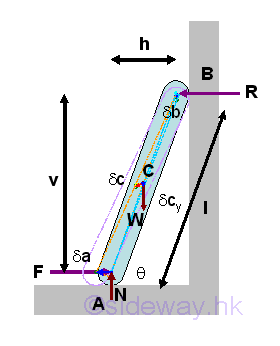Instead of solving the simultaneous equations of complex frame and machine problems, these complex equilibrium can also be determined by applying the principle of virtual work. For example, in the ideal case with smooth wall, the friction force F required to retain the ladder of weight W in position can be determined by the principle of virtual work. Since a virtual counter-clockwise angular displacement along with the angular dimension, θ is in positive sense, the virtual clockwise angular displacement implies a negative sense of the virtual displacements. Therefore the sense of virtual displacements are determined by the virtual angular displacement. ImplyOr, by solving the equilibrium equations of the free body  simultaneously. Imply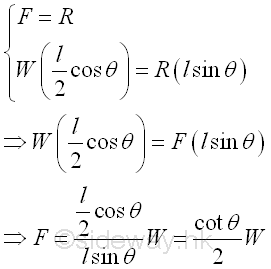Therefore, if the relation of the virtual displacements of the interested forces can be obtained, the interested force can be determined by the principle of virtual work.

## Mechanical Efficiency

In practical mechanical problems, friction forces are always involved. Friction force is a non-conservative force depending on the path travelled. Friction force can be constructive or destructive force. Friction force in a ladder problem is a constructive force, but friction force in the clamping vise problem can be a destructive force. Since work is used  to overcome the work due to the friction force,  the work output of a mechanical device is usually less than the work input to the mechanical device. The mechanical efficiency, η of a mechanical device is defined as the ratio of the output work over the input work to measue the efficiency of a mechanical design for a device or machine. Imply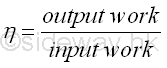For example a toggle vise,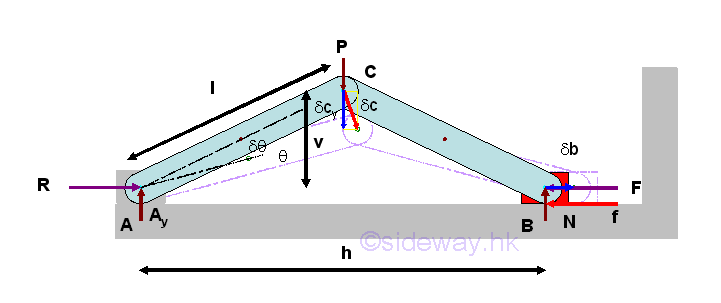The relation between two virtual displacements along the two interested forces can be determined using differential calculus. Geometrically, the positions of points C and B can be expressed in term of the length, l of linkage and the leaning angle, θ. Therefore the virtual differential displacements can be obtained by differentiation. And the relation between two virtual displacements can then be obtained by equating the two relations. ImplyConsider an ideal case, with friction force, f equal to zero. Since a virtual counter-clockwise angular displacement along with the angular dimension, θ is in positive sense, the virtual clockwise angular displacement implies a negative sense of the virtual displacements. Therefore the sense of virtual displacements are determined by the virtual angular displacement. Besides, the two virtual displacement are in opposite sense. Applying the principle of virtual work. ImplyThe mechanical efficiency of the ideal case is equal to one. Imply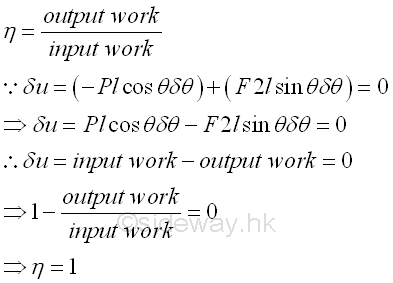Consider a practical case, with friction force, f equal to f. Since a virtual counter-clockwise angular displacement along with the angular dimension, θ is in positive sense, the virtual clockwise angular displacement implies a negative sense of the virtual displacements. Therefore the sense of virtual displacements are determined by the virtual angular displacement. Besides, the two virtual displacement are in opposite sense. Similarly, applying the principle of virtual work. Imply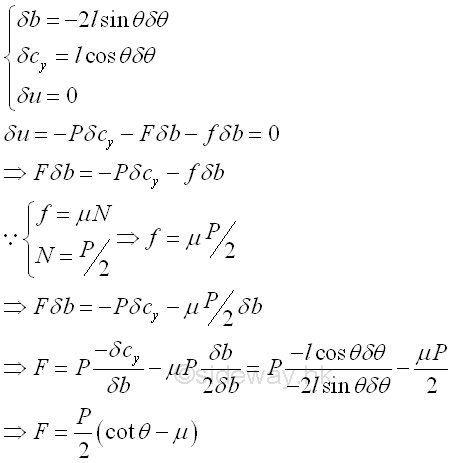The mechanical efficiency of the practical case is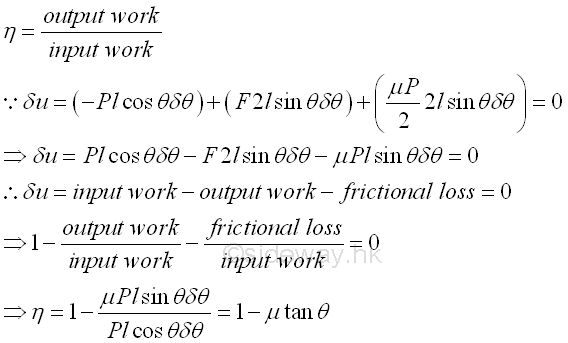When there is no friction al force, μ is equal to zero, the mechanical efficiency, η is equal to 1. And the mechanical efficiency is equal to zero when μtanθ is equal to 1. Therefore the coefficient of friction should be limited to a value such that  μtanθ i is smaller than 1. Imply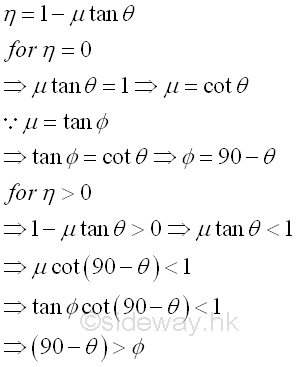ID: 121100089 Last Updated: 12/10/2012 Revision: 0 Ref:References

1. I.C. Jong; B.G. rogers, 1991, Engineering Mechanics: Statics and Dynamics
2. F.P. Beer; E.R. Johnston,Jr.; E.R. Eisenberg, 2004, Vector Mechanics for Engineers: StaticsHome 5

Management

HBR 3

Information

Recreation

Culture

Chinese 1097

English 339

Computer

Hardware 249

Software

Application 213

Latex 52

Manim 205

KB 1

Numeric 19

Programming

Web 289

Unicode 504

HTML 66

CSS 65

SVG 46

ASP.NET 270

OS 429

Python 72

Knowledge

Mathematics

Algebra 84

Geometry 34

Calculus 67

Engineering

Mechanical

Rigid Bodies

Statics 92

Dynamics 37

Control

Natural Sciences

Electric 27